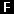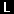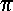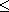Chapter 5 - Functions

Mathematically, a function is a rule for giving a number (the result) in exchange for another (the argument, or operand) & so is really a unary operation. The ZX81 has some of these built into it & their names are the words written under the keys. SQR, for instance, is the familiar square root function, and

PRINT SQR 9

gives 3, the square root of 9. (To get SQR, you first press the FUNCTION key - shifted NEWLINE. This changes the cursor to. Now press the SQR key (H): SQR appears on the screen and the cursor changes back to. The same method works for all the words that are written underneath the keys, almost all of which are function names.)

Try

PRINT SQR 2

You can test the accuracy of the answer by

PRINT SQR 2*SQR 2

which ought to give 2. Note that both SQRs are worked out before the *, and in fact all functions (except one - NOT) are worked out before the five operations +, -, *, / and **. Again, you can circumvent this rule using brackets -

PRINT SQR (2*2)

gives 2.

Here are some functions (there is a complete list in appendix C). If your maths is not up to understanding some of these, it does not matter - you will still be able to use the computer.

 SGN The sign function (sometimes called signum to avoid confusion with SIN). The result is -1, 0 or +1 according as the argument is negative, zero or positive. ABS The absolute value, or modulus. The result is the argument made positive, so that        ABS -3.2 = ABS 3.2 = 3.2 SIN * COS * TAN * ASN arcsin * ACS arccos * ATN arctan * LN natural logarithm (to base 2.718281828459045..., alias e) EXP exponential function SQR square root INT integer part. This always rounds down, so INT 3.9 = 3 & INT -3.9 = -4. (An integer is a whole number, possibly negative.) PI= 3.1415265358979..., the girth in cubits of a circle one cubit across. PI has no argument. (Only ten digits of this are actually stored in the computer, & only eight will be displayed.) RND Neither has RND an argument. It yields a random number between 0 (which value it can take) & 1 (which it cannot).

* The trigonometrical functions. These work in radians, not degrees.

Using the jargon of the last chapter, all these except PI & RND are unary operations with priority 11. (PI & RND are nullary operations, because they have no operands.)

The trigonometrical functions, & EXP, LN & SQR, are generally calculated to 8 digits accuracy.

RND & RAND: These are both on the same key, but whereas RND is a function, RAND is a keyword, like PRINT. RAND is used for controlling the randomness of RND.

RND is not truly random, but follows a fixed sequence of 65536 numbers that happen to be so jumbled up as to appear random (RND is pseudo-random). You can use RAND to start RND off at a definite place in this sequence by typing RAND, & then a number between 1 & 65535, & then NEWLINE. It's not so important to know where a given number starts RND off, as that the same number after RAND will always start RND off at the same place. For instance, type

RAND 1          (& NEWLINE)

& then

PRINT RND

& type both these in turn several times. (Remember to use FUNCTION to get RND.) The answer from RND will always be 0.0022735596, not a very random sequence.

RAND 0

(or you can miss out the 0) acts slightly differently: it judges where to start RND off by how long the television has been on, & this should be genuinely random.

Note: In some dialects of BASIC you must always enclose the arguments of a function on brackets. This is not the case in ZX81 8K BASIC.

Summary

Statement: RAND

Functions: SGN. ABS, SIN, COS, TAN, ASN, ACS, ATN, LN, EXP, SQR, INT, PI, RND

FUNCTION

Exercises

1. To get common logarithms (to base 10), which are what you'd look up in log tables, divide the natural logarithm by LN 10. For instance, to find log 2,

PRINT LN 2/LN 10

Try doing multiplication & division using logs, using the ZX81 as a set of log tables in this way (for antilogs, see chapter 2, exercise 3). Check the answer using * and / - which are easier, quicker, more accurate, & much to be preferred.

2. EXP & LN are mutually inverse functions in the same sense that if you apply one & then the other, you get back to your original number. For instance,

LN EXP 2 = EXP LN 2 = 2

The same also holds for SIN & ASN, for COS & ACS, & for TAN & ATN. You can use this to test how accurately the computer works out these functions.

3.radians are 180°, so to convert from degrees to radians you divide by 180 & multiply by: thus

PRINT TAN (45/180*PI)

gives tan 45° (1).

To get from radians to degrees, you divide by& multiply by 180.

4. Try

PRINT RND

a few times to see how the answer varies. Can you detect any pattern? (Unlikely.)

How would you use RND & INT to get a random whole number between 1 & 6, to represent the throw of a die? (Answer: INT (RND *6) +1.)

5. Test this rule:

Suppose you choose a number between 1 & 872 & type

RAND & then your number (& NEWLINE)

Then the next value of RND will be

(75 * (your number + 1) - 1)/65536

6. (For mathematicians only.)

Let p be a [large] prime, & let a be a primitive root modulo p.

Then if bi is the residue of ai modulo p (1bi < p-1), the sequence

bi-1 / p - 1

is a cylindrical sequence of p-1 distinct numbers in the range 0 to 1 (excluding 1). By choosing a suitably, these can be made to look fairly random.

65537 is a Mersenne prime, 216-1. Use this & Gauss' law of quadratic reciprocity, to show that 75 is a primitive root modulo 65537.

The ZX81 uses p=65537 & a=75, & stores some bi-1 in memory. The function RND involves replacing bi-1 in memory by bi+1-1, & yielding the result (bi+1-1)/(p-1). RAND n (with 165535) makes bi equal to n+1.

7. INT always rounds down. To round to the nearest integer, add 0.5 first. For instance,

 INT (2.9+0.5) = 3 INT (2.4+0.5) = 2 INT (-2.9+0.5) = -3 INT (-2.4+0.5) = -2

Compare these with the answers you get when you don't add 0.5

8. Try

PRINT PI, PI -3, PI -3.1, PI -3.14, PI -3.141

This shows how accurately the computer stores.

Previous: Chapter 4    Next: Chapter 6